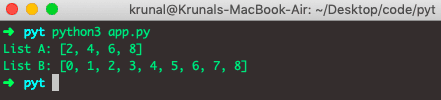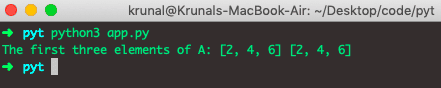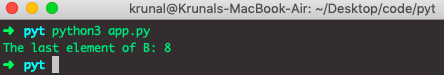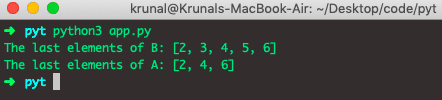# Python Slice Notation: The Complete Guide

Slicing is used to retrieve the subset of values. The basic slicing technique is to define a starting point, the stopping point, and the step size – also known as a stride. This tutorial will show the Python slice notation and how to use it effectively.

## Python Slice Notation

Python slice() is a built-in constructor that creates the slice object representing the set of indices specified by range(start, stop, step). If you want to get specific list values specified by range, then Python slice() is the solution.

First, we will create a list of values.

```# app.py

A = list(range(2,10,2))
B = list(range(9))

print("List A:",A)
print("List B:",B)```

See the output.## Basic Slicing in Python

The extended indexing syntax used for slicing is the following.

### Syntax

`List[start:stop:step]`

The start argument and the step argument both default to none – the only required case is stopped.

Did you notice this is similar to how the range was used to define lists A and B? The slice object represents the indices specified by range(start: stop: step).

It is important to note that the first element is index 0, NOT index 1. This is why we are using two lists for this exercise.

List A’s elements are numbered according to the ordinal position, while List B’s elements are the numbers that would be used to index them ( for the first element 0, etc.).

With extended indexing syntax, we retrieve a range of values.

For example, all values are retrieved with a colon. Now, let’s do some examples.

### Retrieve the first two elements from List A

Let’s get the first three elements of the list using the extended slicing syntax.

```# app.py

A = list(range(2,10,2))
B = list(range(9))

print("The first three elements of A:",A[0:3], A[:3])```

We first have written the A[0:3] in the above code, from index 0 to index 2. So basically, it includes indexes 0, 1, and 2. In our case, it is 2, 4, and 6. So the output would be 2,4,6

In the second case, we have written A[:3]. The first three elements have indexes 0, 1, and 2.

So, the output would be the same as in the first case.### Retrieve the last element of the B List with a negative number

See the following code.

```# app.py

A = list(range(2,10,2))
B = list(range(9))

print("The last element of B:", B[-1])
```

See the output.In the List, the index starts from 0. Also, if we count from the last element of the list, the index starts from -1.

So, on the list, the last element’s index is always -1.

So, in the above example, the last element of List B is 8. Remember that the list starts from 0.

Now, also, let’s take another example and read from the left.

See the following code.

```# app.py

A = list(range(2,10,2))
B = list(range(9))

print("The last elements of B:", B[-7: -2])
print("The last elements of A:", A[-4:-1])
```

See the output.## Understanding slice notation in Python

The summary of slice notation is the following.

```a[start:stop]  # elements start through stop-1
a[start:]      # elements start through the rest of the list
a[:stop]       # elements from the beginning through stop-1
a[:]           # a copy of the whole list```

There is also a step value, which can be used with any of the above.

`a[start:stop:step]`

The critical point to remember is that a: stop value represents the first value that is not in the selected slice.

So, the difference between stop and start is the number of elements chosen (if the step is 1, the default).

The other feature is that start or stop may be a negative number, which means it counts from the end of the array instead of the beginning.

```a[-1]    # last item in the list
a[-2:]   # last two items in the list
a[:-2]   # everything except the last two items```

Similarly, the step may be a negative number.

```a[::-1]    # all items in the list, reversed
a[1::-1]   # the first two items, reversed
a[:-3:-1]  # the last two items, reversed
a[-3::-1]  # everything except the last two items, reversed```

The ASCII art diagram is helpful too for remembering how slices work.

``` +---+---+---+---+---+---+
| k | r | u | n | a | l |
+---+---+---+---+---+---+
0   1   2   3   4   5   6
-6  -5  -4  -3  -2  -1```

That’s it for this tutorial.

### 2 thoughts on “Python Slice Notation: The Complete Guide”

1.Good article, but I need to know what the double :: sign explicitly means? a[::-1] means first : one starts from the beginning list travels to end? then the second :-1 means reverse what ever is before it? I guess. But what does the second : stating ? and what would a[::] mean?

2.